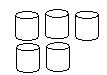Name: ___________________Date:___________________

 Email us to get an instant 20% discount on highly effective K-12 Math & English kwizNET Programs!

### Grade 2 - Mathematics1.46 Working with Numbers to 100 - Review TestName: ___________________Date:___________________

### Grade 2 - Mathematics1.46 Working with Numbers to 100 - Review Test

 Q 1: 6 + 1061516 Q 2: What number comes before 15?14111617 Q 3: 14-9=465 Q 4: Write 8 + 9 in doubles plus one form.9 + 6 + 2 = 178 + 8 + 1 = 177 + 7 + 3 = 176 + 6 + 5 = 17 Q 5: Count the number in the figure and answer even or odd.EvenOdd Q 6: You have 18 erasers. How many dozens do you have?one and one-half dozens16 dozens2 dozens Q 7: Half of 6 is3126 Q 8: In 45, which digit is in the tens place?45 Question 9: This question is available to subscribers only! Question 10: This question is available to subscribers only!